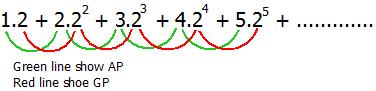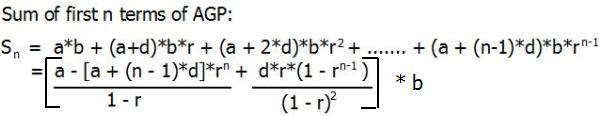# Sum of Arithmetic Geometric Sequence

In mathematics, an arithmetico–geometric sequence is the result of the term-by-term multiplication of a geometric progression with the corresponding terms of an arithmetic progression.is an arithmetico–geometric sequence.

Given the value of a(First term of AP), n(Number of terms), d(Common Difference), b(First term of GP), r(Common ratio of GP). The task is find the sum of first n term of the AGP.

Examples:

```Input : First term of AP, a = 1,
Common difference of AP, d = 1,
First term of GP, b = 2,
Common ratio of GP r = 2,
Number of terms, n = 3
Output : 34
Explanation
Sum = 1*2 + 2*22 + 3*23
= 2 + 8 + 24
= 34
```

## Recommended: Please try your approach on {IDE} first, before moving on to the solution.

The nth term of an arithmetico–geometric sequence is the product of the n-th term of an arithmetic sequence and the nth term of a geometric one. Arithmetico–geometric sequences arise in various applications, such as the computation of expected values in probability theory. For example Counting Expected Number of Trials until Success.

n-th term of an AGP is denoted by: tn = [a + (n – 1) * d] * (b * rn-1)

Method 1: (Brute Force)
The idea is to find each term of the AGP and find the sum.

Below is the implementation of this approach:

## C++

 `// CPP P rogram to find the sum of first n terms. ` `#include ` `using` `namespace` `std; ` ` `  `// Return the sum of first n term of AGP ` `int` `sumofNterm(``int` `a, ``int` `d, ``int` `b, ``int` `r, ``int` `n) ` `{       ` `    ``// finding the each term of AGP and adding ` `    ``// it to sum. ` `    ``int` `sum = 0; ` `    ``for` `(``int` `i = 1; i <= n ; i++)     ` `        ``sum += ((a + (i -1) * d) * (b * ``pow``(r, i - 1)));   ` `    ``return` `sum; ` `} ` ` `  `// Driven Program ` `int` `main() ` `{ ` `    ``int` `a = 1, d = 1, b = 2, r = 2, n = 3; ` `    ``cout << sumofNterm(a, d, b, r, n) << endl; ` `    ``return` `0; ` `}  `

## Java

 `// Java Program to find the sum of first n terms. ` `import` `java.io.*; ` ` `  `class` `GFG { ` `     `  `    ``// Return the sum of first n term of AGP ` `    ``static` `int` `sumofNterm(``int` `a, ``int` `d, ``int` `b, ``int` `r, ``int` `n) ` `    ``{       ` `        ``// finding the each term of AGP and adding ` `        ``// it to sum. ` `        ``int` `sum = ``0``; ` `        ``for` `(``int` `i = ``1``; i <= n ; i++)     ` `            ``sum += ((a + (i -``1``) * d) * (b * Math.pow(r, i - ``1``)));   ` `        ``return` `sum; ` `    ``} ` `     `  `    `  `    ``// Driven Program ` `    ``public` `static` `void` `main(String args[]) ` `    ``{ ` `        ``int` `a = ``1``, d = ``1``, b = ``2``, r = ``2``, n = ``3``; ` `        ``System.out.println(sumofNterm(a, d, b, r, n)); ` `         `  `    ``} ` `} ` ` `  `// This code is contributed by Nikita Tiwari. `

## Python3

 `# Python3 code to find the  ` `# sum of first n terms. ` `import` `math ` ` `  `# Return the sum of first  ` `# n term of AGP ` `def` `sumofNterm( a , d , b , ` `                    ``r , n ): ` `    ``# finding the each term  ` `    ``# of AGP and adding it to sum. ` `    ``sum` `=` `0` `    ``for` `i ``in` `range``(``1``,n``+``1``): ` `        ``sum` `+``=` `((a ``+` `(i ``-``1``) ``*` `d) ``*`  `            ``(b ``*` `math.``pow``(r, i ``-` `1``))) ` `    ``return` `int``(``sum``) ` ` `  `# Driven Code ` `a ``=` `1` `d ``=` `1` `b ``=` `2` `r ``=` `2` `n ``=` `3` `print``(sumofNterm(a, d, b, r, n))  ` ` `  `# This code is contributed by "Sharad_Bhardwaj". `

## C#

 `// C# Program to find the sum of first n terms. ` `using` `System; ` ` `  `class` `GFG { ` `     `  `    ``// Return the sum of first n term of AGP ` `    ``static` `int` `sumofNterm(``int` `a, ``int` `d, ``int` `b, ``int` `r, ``int` `n) ` `    ``{  ` `        ``// Finding the each term of AGP  ` `        ``// and adding it to sum. ` `        ``int` `sum = 0; ` `        ``for` `(``int` `i = 1; i <= n ; i++)  ` `            ``sum += (``int``)((a + (i -1) * d) * ` `                         ``(b * Math.Pow(r, i - 1)));  ` `        ``return` `sum; ` `    ``} ` `     `  `     `  `    ``// Driver Code ` `    ``public` `static` `void` `Main() ` `    ``{ ` `        ``int` `a = 1, d = 1, b = 2, r = 2, n = 3; ` `        ``Console.Write(sumofNterm(a, d, b, r, n)); ` `    ``} ` `} ` ` `  `// This code is contributed by vt_m. `

## PHP

 ` `

Output:

```34
```

Method 2: (Using Formula)Proof,

```Series,
Sn = ab + (a+d)br + (a+2d)br2 + ..... + (a + (n-1)d)brn-1

Multiplying Sn by r,
rSn = abr + (a+d)br2 + (a+2d)br3 + ..... + (a + (n-1)d)brn

Subtract rSn from Sn,
(1 - r)Sn = [a + (a + d)r + (a + 2d)r2 + ...... + [a + (n-1)d]rn-1]
- [ar + (a + d)r2 + (a + 2d)r3 + ...... + [a + (n-1)d]rn]
= b[a + d(r + r2 + r3 + ...... + rn-1)
- [a + (n-1)d]rn]
(Using sum of geometric series Sn  = a(1 - rn-1)/(1-r))
= b[a + dr(1 - rn-1)/(1-r) - [a + (n-1)d]rn]```

Below is the implementation of this approach:

## CPP

 `// CPPP rogram to find the sum of first n terms. ` `#include ` `using` `namespace` `std; ` ` `  `// Return the sum of first n term of AGP ` `int` `sumofNterm(``int` `a, ``int` `d, ``int` `b, ``int` `r, ``int` `n) ` `{ ` `    ``int` `ans = 0;     ` `    ``ans += a;     ` `    ``ans += ((d * r * (1 - ``pow``(r, n-1)))/(1-r));     ` `    ``ans -= (a + (n-1)*d)*``pow``(r, n);     ` `    ``return` `(ans*b)/(1-r); ` `} ` ` `  `// Driven Program ` `int` `main() ` `{ ` `    ``int` `a = 1, d = 1, b = 2, r = 2, n = 3; ` `    ``cout << sumofNterm(a, d, b, r, n) << endl; ` `    ``return` `0; ` `}  `

## Java

 `// Java Program to find the sum of first n terms. ` ` `  `import` `java.io.*; ` `import` `java.math.*; ` ` `  `class` `GFG { ` `     `  `    ``// Return the sum of first n term of AGP ` `    ``static` `int` `sumofNterm(``int` `a, ``int` `d, ``int` `b, ``int` `r, ``int` `n) ` `    ``{ ` `        ``int` `ans = ``0``;     ` `        ``ans += a;     ` `        ``ans += ((d * r * (``1` `- (``int``)(Math.pow(r, n-``1``))))/(``1``-r));     ` `        ``ans -= (a + (n-``1``)*d)*(``int``)(Math.pow(r, n));     ` `        ``return` `(ans*b)/(``1``-r); ` `    ``} ` `      `  `    `  `    ``// Driven Program ` `    ``public` `static` `void` `main(String args[]) ` `    ``{ ` `        ``int` `a = ``1``, d = ``1``, b = ``2``, r = ``2``, n = ``3``; ` `        ``System.out.println(sumofNterm(a, d, b, r, n)); ` `         `  `    ``} ` `} ` ` `  `// This code is contributed by Nikita Tiwari. `

## Python3

 `# Python3 code to find ` `# the sum of first n terms. ` `import` `math ` ` `  `# Return the sum of ` `# first n term of AGP ` `def` `sumofNterm( a , d , b , ` `                    ``r , n ): ` `                         `  `    ``ans ``=` `0` `    ``ans ``+``=` `a ` `     `  `    ``ans ``+``=` `((d ``*` `r ``*` `(``1` `-` `math.``pow``(r, n``-``1``)) ` `                                ``)``/``(``1``-``r)) ` `     `  `    ``ans ``-``=` `(a ``+` `(n``-``1``)``*``d)``*``math.``pow``(r, n) ` `     `  `    ``return` `int``((ans``*``b)``/``(``1``-``r)) ` ` `  `# Driven Code ` `a ``=` `1` `d ``=` `1` `b ``=` `2` `r ``=` `2` `n ``=` `3` `print``(sumofNterm(a, d, b, r, n) )  ` ` `  `# This code is contributed by "Sharad_Bhardwaj". `

## C#

 `// C# Program to find the sum of first n terms. ` `using` `System; ` ` `  `class` `GFG { ` `     `  `    ``// Return the sum of first n term of AGP ` `    ``static` `int` `sumofNterm(``int` `a, ``int` `d, ``int` `b, ``int` `r, ``int` `n) ` `    ``{ ` `        ``int` `ans = 0;  ` `        ``ans += a;  ` `        ``ans += ((d * r * (1 - (``int``)(Math.Pow(r, n-1)))) ` `                                               ``/ (1-r));  ` `        ``ans -= (a + (n-1) * d) * ` `                ``(``int``)(Math.Pow(r, n));  ` `         `  `        ``return` `(ans * b) / (1 - r); ` `    ``} ` `     `  `     `  `    ``// Driver Code ` `    ``public` `static` `void` `Main() ` `    ``{ ` `        ``int` `a = 1, d = 1, b = 2, r = 2, n = 3; ` `        ``Console.Write(sumofNterm(a, d, b, r, n)); ` `    ``} ` `} ` ` `  `// This code is contributed by vt_m. `

## PHP

 ` `

Output:

```34
```

Attention reader! Don’t stop learning now. Get hold of all the important DSA concepts with the DSA Self Paced Course at a student-friendly price and become industry ready.

My Personal Notes arrow_drop_upCheck out this Author's contributed articles.

If you like GeeksforGeeks and would like to contribute, you can also write an article using contribute.geeksforgeeks.org or mail your article to contribute@geeksforgeeks.org. See your article appearing on the GeeksforGeeks main page and help other Geeks.

Please Improve this article if you find anything incorrect by clicking on the "Improve Article" button below.

Improved By : jit_t

Article Tags :
Practice Tags :

Be the First to upvote.

Please write to us at contribute@geeksforgeeks.org to report any issue with the above content.